### Day 22 - Implicit Differentiation - 09.16.15

Update

Bell Ringer

Note: Some of these are problems you have not seen yet.
Find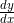using the derivative operator.
Hint: Try to get y by itself on one side of the equation (if you can).

1.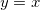1. x

2. y

3. 1

4. 0

5. none of the above

2.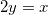1.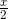2.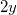3. 2

4. 0

5. none of the above

3.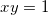1.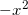2.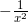3.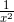4.5. none of the above

4.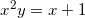1.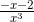2.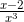3.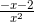4.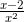5. none of the above

5.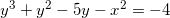1.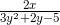2.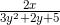3.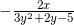4.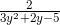5. none of the above

Review
• Pre-calculus
• Slope
• Equation of a Line
• Secant Line vs. Tangent Line (video)
• Tangent Line
• How can the slope of one point be found?
• Finding the derivative of polynomials using limits (example)
• Basic Differentiation Rules
• How can derivatives be calculated using basic differentiation rules?

Lesson
• Challenge 6
• Find the derivative of the following:
•• Hints will be given as needed.
• No computers allowed!

Exit Ticket
• Posted on the board at the end of the block.

Homework
• N/A

Lesson Objectives
• How can implicit differentiation be used to find derivatives?

#### In-Class Help Requests

Standard(s)
• APC.5
• Investigate derivatives presented in graphic, numerical, and analytic contexts and the relationship between continuity and differentiability.
• The derivative will be defined as the limit of the difference quotient and interpreted as an instantaneous rate of change.
• APC.6
• ​The student will investigate the derivative at a point on a curve.
• Includes:
• finding the slope of a curve at a point, including points at which the tangent is vertical and points at which there are no tangents
• using local linear approximation to find the slope of a tangent line to a curve at the point
• ​defining instantaneous rate of change as the limit of average rate of change
• approximating rate of change from graphs and tables of values.
• APC.9
• Apply formulas to find derivatives.
• Includes:
• derivatives of algebraic, trigonometric, exponential, logarithmic, and inverse trigonometric functions
• derivations of sums, products, quotients, inverses, and composites (chain rule) of elementary functions
• derivatives of implicitly defined functions